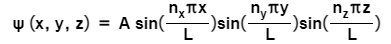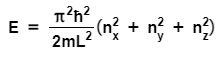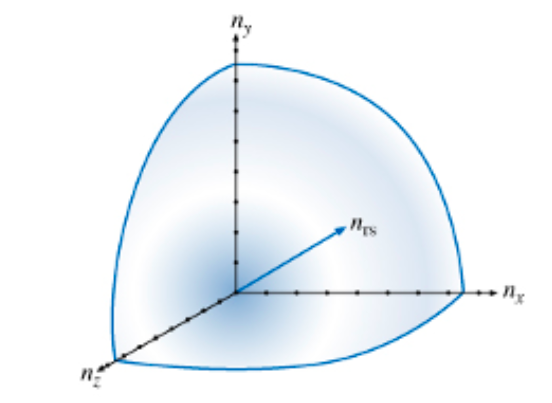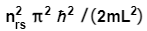# Problem: Problems that require solving the three-dimensional Schrödinger equation can often be reduced to related one-dimensional problems. An example of this would be the particle in a cubical box.Consider a cubical box with rigid walls (i.e., U(x,y,z)=?outside of the cube) and edges of length L. The general solution for this problem iswhere nx, ny, and nz are all positive integers. Note that this solution is just the product of three solutions to the one-dimensional particle in a box. The energy corresponding to the three-dimensional solution is just the sum of the energies for each of the three one-dimensional solutions:Part AWhat is the smallest allowed energy E0 for a particle in a cubical box?Express your answer in terms of ?, m, and L.Part BIf you are dealing with a very large nrs, you can assume that each state (point with integer coordinates) corresponds roughly to one unit of volume inside of the sphere. So, the number of states is approximately equal to the volume of the octant of the sphere. Use this idea to find the number N of states with energy less than or equal to  for a large nrs.Express your answer in terms of nrs.

###### FREE Expert Solution

For a cubic box, the energy  is:

$\overline{){{\mathbf{E}}}_{{\mathbf{n}}}{\mathbf{=}}\frac{{\mathbf{ћ}}^{\mathbf{2}}{\mathbf{\pi }}^{\mathbf{2}}}{\mathbf{2}\mathbf{m}{\mathbf{L}}^{\mathbf{2}}}{\mathbf{\left(}}{{{\mathbf{n}}}_{{\mathbf{x}}}}^{{\mathbf{2}}}{\mathbf{+}}{{{\mathbf{n}}}_{{\mathbf{y}}}}^{{\mathbf{2}}}{\mathbf{+}}{{{\mathbf{n}}}_{{\mathbf{z}}}}^{{\mathbf{2}}}{\mathbf{\right)}}}$, where nx = 1, 2, 3, ......, ny = 1, 2, 3, ...., and nz =1, 2, 3, ....

For a very large nrs:

$\overline{){\mathbf{E}}{\mathbf{=}}\frac{{{\mathbf{n}}_{\mathbf{r}\mathbf{s}}}^{\mathbf{2}}{\mathbf{ћ}}^{\mathbf{2}}{\mathbf{\pi }}^{\mathbf{2}}}{\mathbf{2}\mathbf{m}{\mathbf{L}}^{\mathbf{2}}}}$

79% (367 ratings)###### Problem Details

Problems that require solving the three-dimensional Schrödinger equation can often be reduced to related one-dimensional problems. An example of this would be the particle in a cubical box.

Consider a cubical box with rigid walls (i.e., U(x,y,z)=?outside of the cube) and edges of length L. The general solution for this problem iswhere nx, ny, and nz are all positive integers. Note that this solution is just the product of three solutions to the one-dimensional particle in a box. The energy corresponding to the three-dimensional solution is just the sum of the energies for each of the three one-dimensional solutions:Part A
What is the smallest allowed energy E0 for a particle in a cubical box?

Express your answer in terms of ?, m, and L.

Part B

If you are dealing with a very large nrs, you can assume that each state (point with integer coordinates) corresponds roughly to one unit of volume inside of the sphere. So, the number of states is approximately equal to the volume of the octant of the sphere. Use this idea to find the number N of states with energy less than or equal tofor a large nrs.

Express your answer in terms of nrs.

Frequently Asked Questions

What scientific concept do you need to know in order to solve this problem?

Our tutors have indicated that to solve this problem you will need to apply the Quantum Mechanics concept. You can view video lessons to learn Quantum Mechanics. Or if you need more Quantum Mechanics practice, you can also practice Quantum Mechanics practice problems.